﻿ Text/Equation Boxes

# Text/Equation Boxes

Top  Previous  Next

# Basic Usage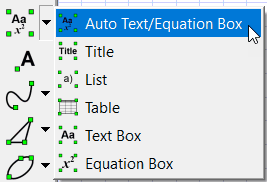1.        Select the Automatic Text/Equation Box tool

2.        Click on the drawing canvas where you want the text box to appear.

3.        Start typing!

As soon as you have clicked, the text toolbar will appear at the bottom of the screen.  This is where you enter and edit the text you want.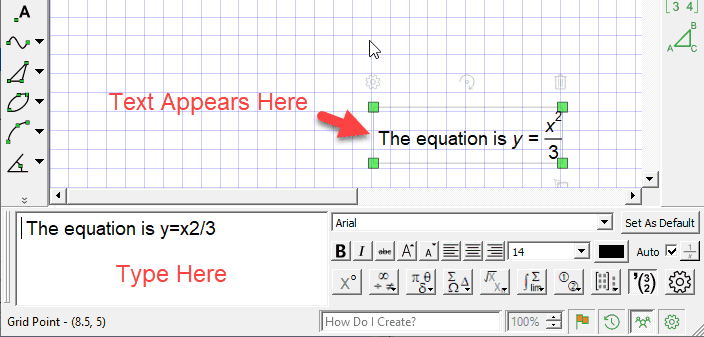Video - Text Boxes

# Text Formatting

The Text toolbar contains basic formatting controls that are similar to those in most word processors.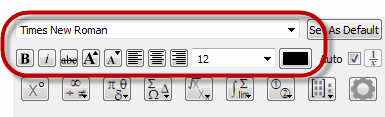Select some text and use the controls to change text size, color and formatting.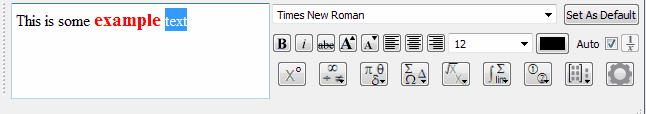# FX Autoquate

You may have noticed something special about our first example - FX Draw had done something special with some of the text we typed.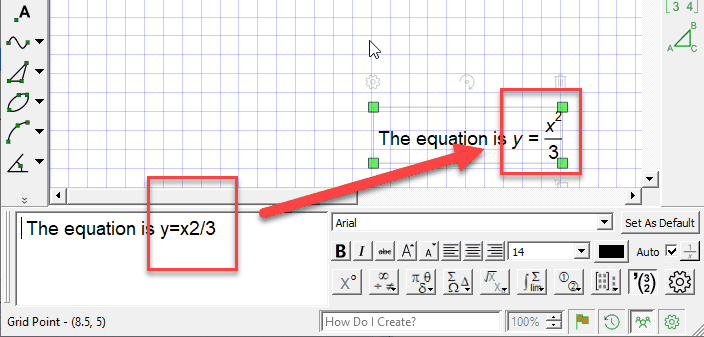We call this new technology "FX Autoquate" - a system which looks at what you have typed and automatically finds the bits which should be formatted as equations (using our traditional FX Equation technology).  YOU don't have to do anything except type!  This new feature can massively improve your productivity when typing mathematics.

FX Autoquate is pretty good and will almost always find the bits that need to be automatically made into equations.  Sometimes you will want to override its suggestions.  You can do this by removing the check mark from the Auto box.  Once this is removed, you can manually mark which bits of your typing you want interpreted as an equation.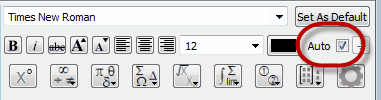# Manually Marking Equations

When you turn off FX Autoquate, you need to manually mark sections of your text as equations.  You do this by marking the text and pressing the 1/x button.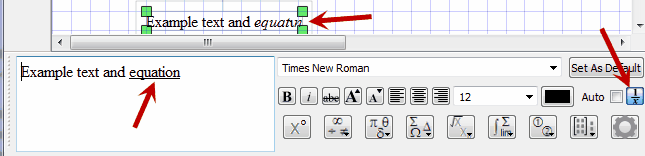You should notice that Auto is off and the 1/x button is depressed.  In the text entry section, the word "equation" has been underlined which is how FX Draw shows you that it is going to interpret that section as an equation.  Your should also notice that, on the canvas, the word "equation" has been rather strangely formatted (including replacing io with the Greek letter io).

Manually marking the equations allows you to produce just about anything.

# Plain Text Boxes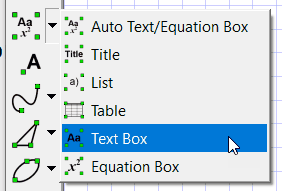If you know that what you are about to type definitely should be interpreted as plain text, choose a Text box rather than an automatic text box.  What you get is exactly the same, it is just that Auto is turned off and the 1/x button has not been pushed.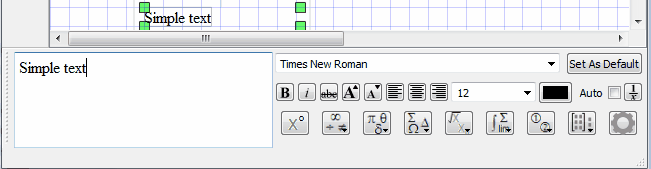This can be particularly useful if you want to type an ordered pair and FX Autoquate keeps turning them into column vectors.

Plain Text boxes can also be useful if FX Autoquate is consistently misinterpreting something you are often typing. PLEASE TELL US if this occurs.  We are always looking to improve the accuracy of FX Autoquate!

# Equation Boxes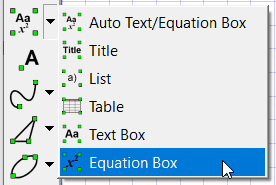If you know that what you are about to type definitely should be interpreted as an equation, choose an Equation box rather than an automatic text box.  What you get is exactly the same, it is just that Auto is turned off and the 1/x button has been pushed so anything you type will definitely be interpreted as an equation.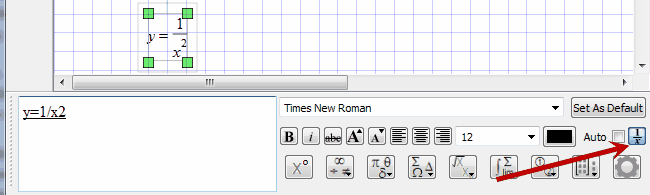FX Draw shares its equation tool with FX Equation. A full discussion on how to enter equations using the equation tool is available in Entering Equations.Video - Introduction To Equations

# Equation Toolbar

FX Equation has long had a toolbar to make entering some of the more esoteric equations a bit easier.  FX Draw  provides you with the same functionality.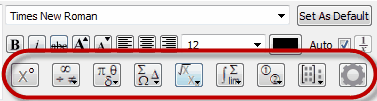Each button provides you with access to FX Equation features.  You never HAVE to use the buttons.  All equations can be entered solely using the keyboard as discussed in Entering Equations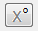Enters a degree sign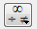Allows entry of most mathematical symbols.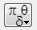Allows entry of lower case Greek letters.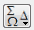Allow entry of upper case Greek letters.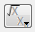Allows the creation of many mathematical expressions.  Usually the current selection is affected.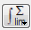Allow for the creation of more complicated mathematical constructs such as limits, integrals and summation.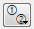Allows you to create numbers in circles which are useful for simultaneous equations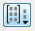Matrices Vectors and Piecewise defined functions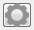Change the equation options.

# Equation Contexts

In May 2019, we added a new concept to the equation tool - equation contexts.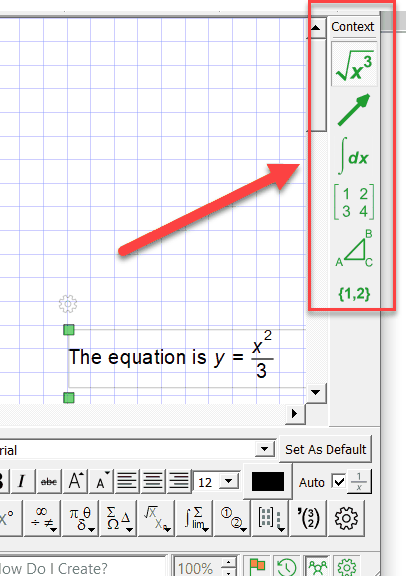# What Are Equation Contexts?

Equation contexts are a quick way to tell FX Equation the type of equation you are working on at the moment. This allows FX Equation to set up its options to suit that type of equation. For example, choosing the Vector context...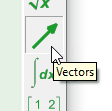sets up the equation tool so that typing commas produces column vectors; typing double capitals adds an arrow above and the tool will take its best guess at lower case vectors.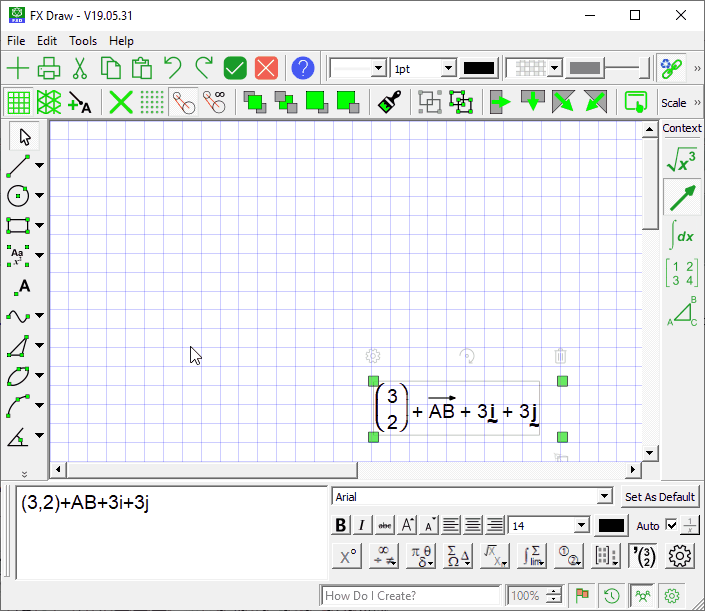There are six contexts available:

 • General Algebra (used for most equations).
 • Vectors.
 • Calculus.
 • Matrices.
 • Geometry.
 • Sets.